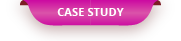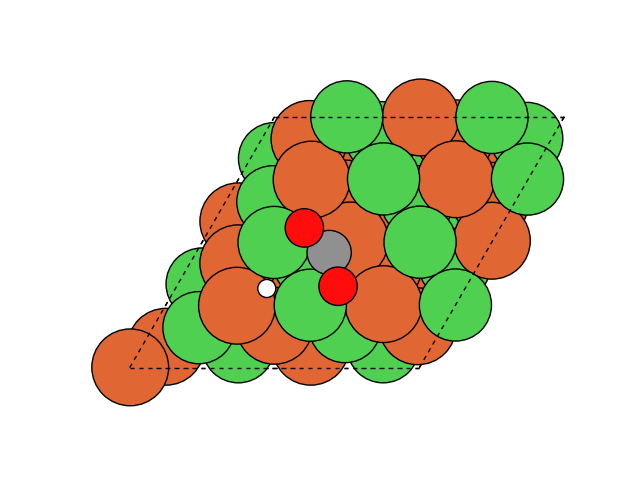A molecular dynamics simulation of the catalytic material was performed using a general-purpose graph neural network ...

# General-purpose graph Molecular dynamics simulation of catalytic materials using neural network force fields

A molecular dynamics simulation of the catalytic material was performed using a general-purpose graph neural network force field (GNN force field). The GNN force field used was developed by the Open Catalyst Project sponsored by Facebook and Carnegie Mellon University, and it is a learning data of about 130 million first-principles calculation results targeting catalytic materials. It is constructed as a molecular dynamics calculation of a wide range of catalytic materials. In this case, we analyzed the CO2 methanation reaction using a Ni-based catalyst.

#### Calculation model / calculation conditions

The CO 2 methanation reaction formula is CO 2 + 4H 2 → CH 4 + 2H 2 O, but here, as shown in the reaction process below, hydrogen molecules are dissociated and adsorbed on the catalyst surface to become hydrogen atoms, and CO 2 molecules It is assumed that it progresses by adding hydrogen to.

The catalyst model was the Ni (111) plane, and the case where a part of the Ni atom was replaced with Mg and Fe was also examined. Figure 1 shows the calculation model at the start of the reaction. The Nudged elastic band (NEB) method was used to calculate the chemical reaction. The NEB method is a method for searching the transition state from the structure of the start state and the end state of the reaction. The GNN used was Dimnet ++.

#### Calculation result

Figure 2 shows the animation of the methanation reaction obtained from the NEB calculation. It can be seen that as hydrogenation progresses, water molecules are desorbed and finally methane molecules are produced. Performing such NEB calculation using first-principles calculation requires a huge amount of calculation time, but here, the time required for NEB calculation in one reaction process is only a few minutes, and the calculation is very fast. It is finished.